﻿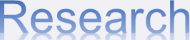Algebra and Number Theory The theory of Lie groups, Lie algebras and their representations play an important role in many of the recent development of mathematics and in the interaction of mathematics with physics. Our research includes representation theory of reductive groups, Kac-Moody algebras, quantum groups, and conformal field theory. Number theory has a long and distinguished history, and the concepts and problems relating to the theory have been instrumental in the foundation of a large part of mathematics. Number theory has flourished in recent years, as made evident by the proof of Fermat's Last Theorem. Our research specializes in automorphic forms.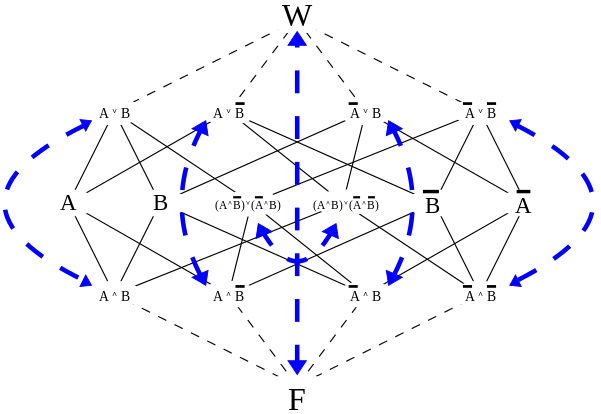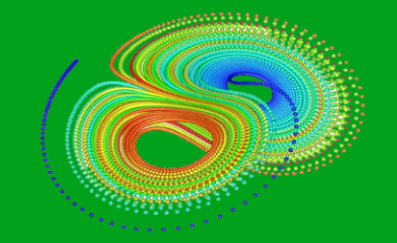Analysis and Differential Equations The analysis of real and complex functions plays fundamental role in mathematics. This is a classical yet still vibrant subject that has a wide range of applications. Differential equations are used to describe many scientific, engineering and economic problems. The theoretical and numerical study of such equations is crucial in understanding and solving problems. Our research areas include complex analysis, exponential asymptotics, functional analysis, nonlinear equations and dynamical systems, and integrable systems. Geometry and Topology Geometry and topology provide an essential language describing all kinds of structures in nature. The subject has been vastly enriched by close interaction with other mathematical fields and with various fields of science such as physics, astronomy and mechanics. The result is the great advances in the subject, as highlighted by the proof of the Poincaréonjecture. The active research areas in the department include algebraic geometry, differential geometry, low-dimensional topology, equivariant topology, combinatorial topology, and geometrical structures in mathematical physics.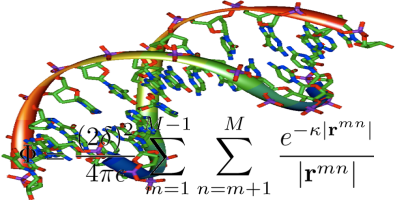Applied and Computational Mathematics The applications of mathematics to interdisciplinary science areas include: material science, multiscale modeling, mutliphase flows, evolutionary genetics, environmental science, numerical weather prediction, ocean and coastal modeling, astrophysics and space science. The focus is on the development of advance algorithms and efficient computational schemes. Current research areas include: parallel algorithms, heterogeneous network computing, graph theory, image processing, computational fluid dynamics, singular problems, adaptive grid method, non-equilibrium flow simulations. Probability and Statistics Statistics, the science of collecting, analysing, interpreting, and presenting data, is an essential tool in a wide variety of academic disciplines as well as for business, government, medicine and industry. Our research is conducted in four categories. In time series and dependent data, we study inference from nonstationarity, nonlinearity, long-memory behavior, and continuous time models. In resampling methodology, we concentrate on block bootstrap, bootstrap for censored data, and Edgeworth and saddle point approximations. In stochastic processes and stochastic analysis, we investigate filtering, diffusion and Markov processes, and stochastic approximation and control. In survival analysis, we estimate the survival function and errors in variables for general linear models. Current research in Probability includes limit theory.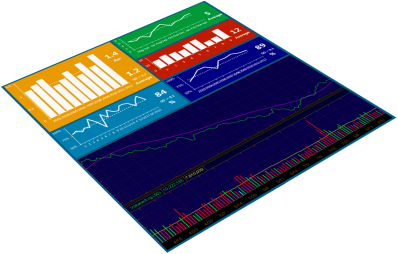Financial Mathematics Financial mathematics is one of the fastest growing research fields in applied mathematics. International banking and financial firms around the globe are hiring science PhDs who can use advanced analytical and numerical techniques to price financial derivatives and manage portfolio risks. The trend has been accelerating in recent years on numerous fronts, driven both by substantial theoretical advances as well as by a practical need in the industry to develop effective methods to price and hedge increasingly complex financial instruments. Current research areas include pricing models for exotic options, the development of pricing algorithms for complex financial derivatives, credit derivatives, risk measures for banking regulation, credit and funding value adjustment, risk management, stochastic analysis of interest rates and related models, econometric analysis of option pricing models, real estate derivative pricing, and stock market microstructure analysis.